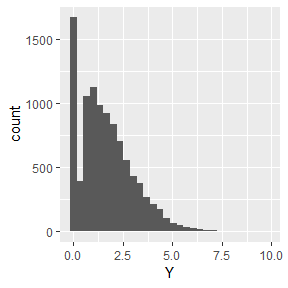# Tweedie Distribution

## Introduction

The tweedie distribution is a relatively new comer to generalized linear model framework. It has three parameters, $$\mu$$, $$\sigma^2$$, $$\rho$$, and has a number of unique characteristics. Many other distributions are special cases of the tweedie distribution.

• Distributions
• $$\rho$$ = 0 gaussian
• $$\rho$$ = 1 poisson
• $$\rho$$ = 2 gamma
• $$\rho$$ = 3 inverse gaussian

The probability density function cannot be evaluated directly. Instead special algorithms must be created to calculate the density. Interested statisticians should read Evaluation of Tweedie exponential dispersion model densities by Fourier inversion. The author created the R package tweedie; which GlmSimulatoR relies upon.

Due to computational demands associated with the tweedie distribution and software available in R, this package focuses on tweedie distributions with $$\rho$$ in [1, 2] and $$\sigma^2$$ equal to 1.

## Building a glm model

To test out R’s special glm function for the tweedie distribution, lets generate data and see how close our estimates are. We will be using the cplm package.

library(GlmSimulatoR)
library(ggplot2)
library(cplm, quietly = TRUE)
set.seed(1)

simdata <- simulate_tweedie(weight = .2, ancillary = 1.15, link = "log")

ggplot(simdata, aes(x = Y)) +
geom_histogram(bins = 30)The response variable is usually zero, but sometimes it is positive. This is what makes the tweedie distribution unique.

glmModel <- cpglm(Y ~ X1, data =simdata, link = "log")
summary(glmModel)
##
## Call:
## cpglm(formula = Y ~ X1, link = "log", data = simdata)
##
## Deviance Residuals:
##     Min       1Q   Median       3Q      Max
## -1.9832  -0.8648  -0.1652   0.5337   4.0653
##             Estimate Std. Error t value Pr(>|t|)
## (Intercept)  0.21157    0.04286   4.936 8.09e-07 ***
## X1           0.19587    0.02780   7.047 1.95e-12 ***
## ---
## Signif. codes:  0 '***' 0.001 '**' 0.01 '*' 0.05 '.' 0.1 ' ' 1
##
## Estimated dispersion parameter: 1.0057
## Estimated index parameter: 1.1521
##
## Residual deviance: 11829  on 9998  degrees of freedom
## AIC:  33421
##
## Number of Fisher Scoring iterations:  5

The estimated index parameter is very close to $$\rho$$, and the estimated slope is close to $$\beta$$. Overall the function worked well.